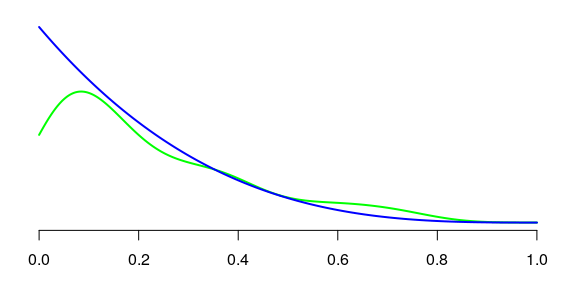Want to share your content on R-bloggers? click here if you have a blog, or here if you don't.

It seems to me that the `kde1d` package (One-Dimensional Kernel Density Estimation) is not very known. I’ve never heard of it on Stack Overflow, except in an answer of mine.

However this is a great package, IMHO. I’m going to show why I like it.

### The `d/p/q/r` family

Estimating a density with the `kde1d` function returns a `kde1d` object, and this makes available the density, the distribution function, the quantile function associated to the density estimate, as well as a sampler from the estimated distribution.

Let’s fit a density with `kde1d` to a simulated Gaussian sample:

```library(kde1d)
set.seed(666)
y <- rnorm(100)
fit <- kde1d(y)```

Here is the density estimate, in green, along with the true density, in blue:

```opar <- par(mar = c(3, 1, 1, 1))
plot(NULL, xlim = c(-3.5, 3.5), ylim = c(0, 0.4), axes = FALSE, xlab = NA)
axis(1, at = seq(-3, 3, by=1))
curve(dkde1d(x, fit), n = 300, add = TRUE, col = "green", lwd = 2)
curve(dnorm(x), n = 300, add = TRUE, col = "blue", lwd = 2)```The density can even be evaluated outside the range of the data:

```print(dkde1d(max(y)+1, fit))
##  0.001684873```

The corresponding cumulative distribution function:

```opar <- par(mar = c(4.5, 5, 1, 1))
plot(NULL, xlim = c(-3.5, 3.5), ylim = c(0, 1), axes = FALSE,
xlab = "x", ylab = expression("Pr("<="x)"))
axis(1, at = seq(-3, 3, by=1))
axis(2, at = seq(0, 1, by=0.25))
curve(pkde1d(x, fit), n = 300, add = TRUE, col = "green", lwd = 2)
curve(pnorm(x), n = 300, add = TRUE, col = "blue", lwd = 2)```The corresponding inverse cumulative distribution function is evaluated by `qkde1d`, and `rkde1d` simulates from the estimated distribution.

### Bounded data

By default, the data supplied to the `kde1d` function is assumed to be unbounded. For bounded data, use the `xmin` and/or `xmax` options.

### Estimating monotonic densities

Now, something I use to convince my folks that `kde1d` is great. Consider a distribution having a monotonic density. The base function `density` does not correctly estimate the density (at least, with the default settings):

```set.seed(666)
y <- rbeta(100, 1, 4)
opar <- par(mar = c(3, 1, 1, 1))
plot(NULL, xlim = c(0, 1), ylim = c(0, 4), axes = FALSE, xlab = NA)
axis(1, at = seq(0, 1, by=0.2))
lines(density(y, from = 0, to = 1), col = "green", lwd = 2)
curve(dbeta(x, 1, 4), n = 300, add = TRUE, col = "blue", lwd = 2)```The monotonic aspect of the density does not occur in the estimated density. With `kde1d`, it does:

```fit <- kde1d(y, xmin = 0, xmax = 1)
opar <- par(mar = c(3, 1, 1, 1))
plot(NULL, xlim = c(0, 1), ylim = c(0, 4), axes = FALSE, xlab = NA)
axis(1, at = seq(0, 1, by=0.2))
curve(dkde1d(x, fit), n = 300, add = TRUE, col = "green", lwd = 2)
curve(dbeta(x, 1, 4), n = 300, add = TRUE, col = "blue", lwd = 2)```